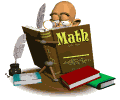# MATH HOMEWORK HELP

•We are currently studying Math Module 4: adding tens and ones and showing how dimes a pennies connect with tens and ones.

Students should know:

the value of a penny and be able to recognize the head and tail

the value of a dime and recognize the head and tail

the terms: greater than, less than, equal to, one more, ten more, one less, ten less

how to use quick tens and ones, draw a two digit number

add and subtract two digit numbers

know the strategy of the "arrow way"; tape drawings

know that the sum of a collection of coins is not the total number of coins, but the value of the coins

be able to compare two numbers

rewrite numbers in the correct sequence and fill in missing numbers

find the mystery number (unknown number) whereever the position is in the equation, especially paying attention to where the equal sign is in the equation

https://www.youtube.com/watch?v=rLZbniMGAVA  video on greater than, less than, equal to

https://www.youtube.com/watch?v=rBkwSl8Tj98  how to know which way the comparative sign goes